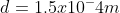High School Physics : Electricity and Magnetism

Example Questions

Example Question #6 : Understanding Electricity

A 4.5V battery is connected to a bulb whose resistance is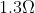. How many electrons leave the battery per minute?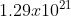electrons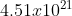electrons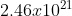electrons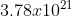electronselectrons

Explanation:

We first need to determine the current coming through the bulb. We can use Ohm’s law to determine this.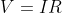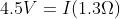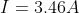Current by definition is the amount of charge per unit time.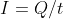We are looking at the number of electrons in one minute which is 60 seconds.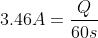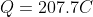We now know the amount of charge passing through in 60 seconds.  We know that the charge of the electron is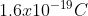.  We can use this to figure out how many electrons are going through.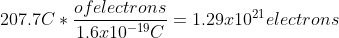Example Question #7 : Understanding Electricity

Nothing happens when birds land on a power line, yet we are warned not to touch a power line with a ladder. What is the difference?

Dangerous current comes from the ground only

There is little to no voltage drop between a bird's two feet, but there is a significant voltage drop between the top of a ladder touching a power line and the bottom of the ladder on the ground

Most birds don’t understand the situation

Birds have extremely high internal resistance compared to humans

There is little to no voltage drop between a bird's two feet, but there is a significant voltage drop between the top of a ladder touching a power line and the bottom of the ladder on the ground

Explanation:

Electricity travels from the point of highest potential to the lowest potential. We measure the difference between these two potentials as the voltage. Since both of the birds' feet are on the wire, both feet have the same potential. However, if the ladder touches the power line and the bottom of the ladder is on the ground, there is a much higher potential difference (as the ground is at 0 potential).  Therefore the electricity will travel down the ladder!

Example Question #8 : Understanding Electricity

When a light switch is turned on, the light comes on immediately because

The electrons coming from the power source move through the initially empty wires very fast

The electricity bill is paid. The electric company can make it take longer when the bill is unpaid

The electrons already in the wire are instantly “pushed” by the voltage difference

The lightbulb may be old with low resistance. It would take longer if the bulb were new and had high resistance

The electrons already in the wire are instantly “pushed” by the voltage difference

Explanation:

It's kind of like when you turn on the faucet; the water doesn't have to travel from the water tower to your house before it starts flowing. Similarly, the electrons are always in the circuit. Once the switch is turned on, there is a voltage difference present, which is what then pushes these electrons through the circuit (like turning on the faucet).

Example Question #13 : Resistors

Ten resistors, each with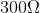resistance, are set up in series. What is their equivalent resistance?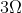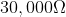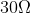Explanation:

For resistors aligned in series, the equivalent resistance is the sum of the individual resistances.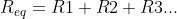Since all the resistors in this problem are equal, we can simplify with multiplication.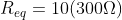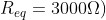Example Question #941 : High School Physics

What is the total resistance of a parallel circuit with resistors of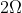,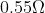, and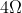?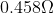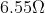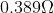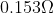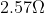Explanation:

The formula for resistance in parallel is: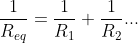We are given the values for each individual resistor, allowing us to solve for the total resistance.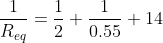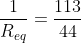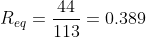Example Question #15 : Resistors

An electric circuit is set up in series with five resistors. If the resistors remain the same, but the circuit is now set up with the resistors in parallel, how would this affect the total resistance?

We need to know the numerical values to solve

Resistance would increase substantially

Resistance would decrease substantially

Resistance would remain unchanged

Resistance would decrease substantially

Explanation:

Think of resistors as doors, preventing the flow of people (electrons). Imagine the following scenarios: a large group of people are in a room and all try to leave at once. If the five resistors are in series, that's like having all of these people trying to go through all five doors before they can leave. In a circuit, all the electrons in the current mast pass through every resistor.

If the resistors are in parallel, it's like having five separate doors from the room. All of a sudden, the group can leave MUCH faster, encountering less resistance to their flow out of the room. The path of the electrons can split, allowing each particle to pass through only one resistor.

From a formula perspective, the resistors in series are simply summed together to find the equivalent resistance.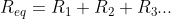In parallel, however, the reciprocals are summed to find the reciprocal equivalent resistance.Adding whole numbers will always give you a much greater result than adding fractions. For the exact same set of resistors, arrangement in series will have a greater total resistance than arrangement in parallel.

Example Question #16 : Resistors

When current in a circuit crosses a resistor, energy is lost. What form does this lost energy most commonly take?

The energy is converted into sound

The energy is converted into motion

The energy is converted into heat

The energy is not converted; it simply disappears

The energy is converted into light

The energy is converted into heat

Explanation:

In basic resistors, energy lost due to resistance is converted into heat. In some cases, other conversions also take place (such as generation of light in a lightbulb), but heat is still dissipated along with any alternative conversations. Lightbulbs, batteries, and other types of resistors will become hot as current passes through them.

Example Question #17 : Resistors

You have a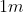long,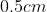diameter copper wire that has an electric current running through it. Which of the following would decrease the wire's overall resistivity?

Increasing the length of the wire

Decreasing the length of the wire

None of these

Decreasing the diameter of the wire

Increasing the diameter of the wire

None of these

Explanation:

The formula for resistance is: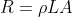Above,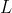is length,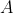is the cross-sectional area of the wire, and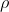is the resistivity of the material, and is a property of that material. The resistivity is constant for a given material, and thus cannot be changed by altering the dimensions of the wire.

Example Question #21 : Resistors

Two aluminum wires have the same resistance.  If one has twice the length of the other, what is the ratio of the diameter of the longer wire to the diameter of the shorter wire?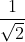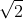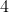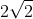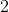Explanation: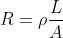The area of a wire is the area of a circle.  So let’s substitute that into our equation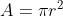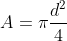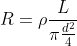This can be simplified to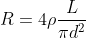Since we know that both resistors have the same resistivity and the same resistance, we can set these equations equal to each other.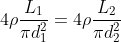Many things fallout which leaves us with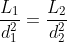We know that the second wire is twice the length as the first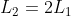So we can substitute this into our equation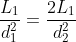The length of the wire drops out of the equation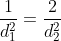Now we can solve for the diameter of the longer wire.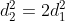Take the square root of both sides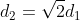Therefore the ratio of the long to the short wire is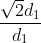OrExample Question #22 : Resistors

What is the diameter of a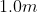length of tungsten wire whose resistance is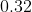ohms?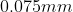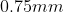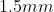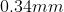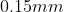Explanation:

We will use the resistivity equation to solve for this.  We know

Length =Resistance =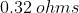ρ of Tungsten =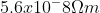The equation for resistivity isWe can rearrange this equation to solve for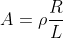In this case the area of the wire is the area of a circle which is equal to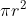We can rearrange this to get the radius by itself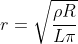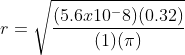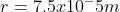To find the diameter we need to multiply this value by 2.